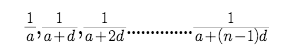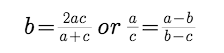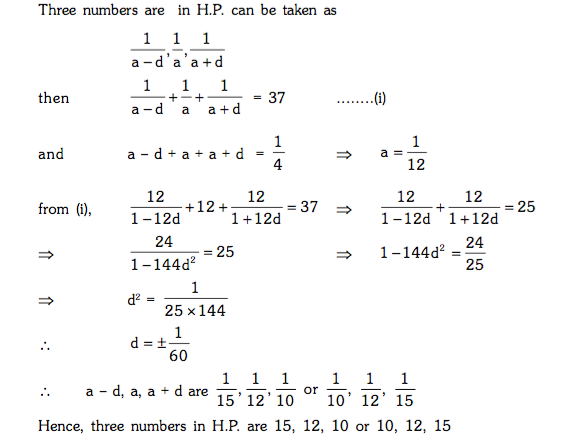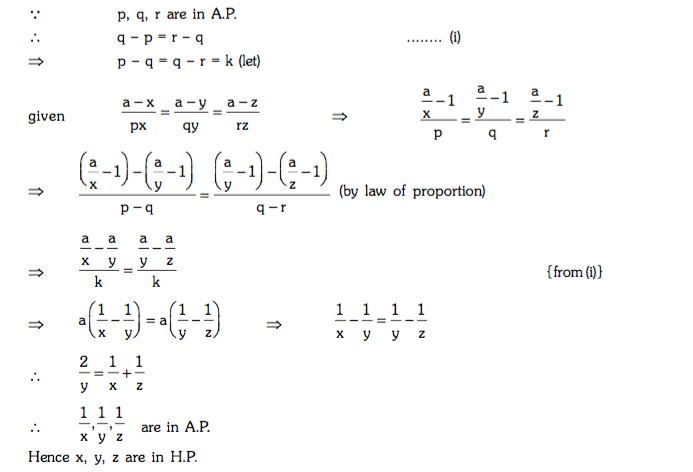# HARMONIC PROGRESSION (HP) - Concept and Properties with Example

HARMONIC PROGRESSION (H.P.)

A sequence is said to be in H.P. if the reciprocal of its terms are in AP.

If the sequence a1, a2, a3, ......., an is an HP then 1/a1, 1/a2,........., 1/an is an AP . Here we do not have the formula for the sum of the n terms of an HP.

The general form of a harmonic progression isProperties :

1. No term of any H.P. can be zero.
2. If a, b, c are in HP, thenExample :

The sum of three numbers are in H.P. is 37 and the sum of their reciprocals is 4 . Find the numbers.

Solution :Example :

Suppose a is a fixed real number such that a-x/px =a-y/qy = a-z/rz

If p, q, r are in A.P., then prove that x, y, z are in H.P.

Solution :Post By : Preeti Rai 20 Apr, 2019 5196 views Maths